### Atoms And Molecules Class 9th Physics & Chemistry AP Board Solution

##### Question 1.Draw the diagram to show the experimental setup for the law of conservation of mass.Answer:Step 1: Take a flask and take a mixture of sodium hydroxide solution and copper sulphate solution.Step 2: Weight the flask and note it.Step 3: Now mix the whole mixture thoroughly.Step 4: Now, weight the flask.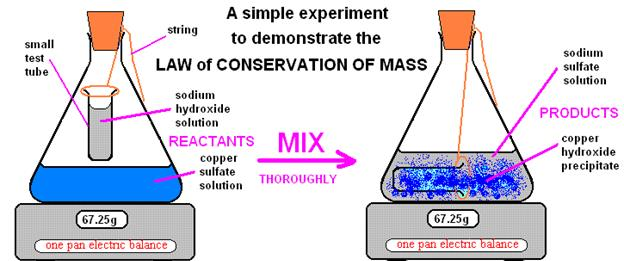We will observe that after mixing there is no change in the mass. This explains the law of conservation of mass.Question 2.Explain the process and precautions in verifying law of conservation of mass.Answer:Law of conservation of mass states that “Matter is neither created nor destroys in a chemical reaction. More simply, the mass of products is equal to the mass of reactants in a chemical reaction.Question 3.15.9g. of copper sulphate and 10.6g of sodium carbonate react together to give 14.2g of sodium sulphate and 12.3g of copper carbonate. Which law of chemical combination is obeyed? How?Answer:Law of conservation of mass is obeyed.Explanation: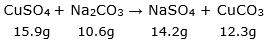Total mass of reactants = 15.9g + 10.6g = 26.5gTotal mass of products = 14.2g + 12.3g = 26.5gAs there is no change in the mass of reactants and products, hence law of conservation is obeyed.Question 4.Carbon dioxide is added to 112g of calcium oxide. The product formed is 200g of calcium carbonate. Calculate the mass carbon dioxide used. Which law of chemical combination will govern your answer.Answer:The reaction taking place is: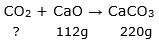Let the mass of CO2 is xg.According to the law of conservation of mass, the mass of reactants is equal to the mass of products.Therefore,⇒ x + 112g = 220g⇒ x = 220g – 112g⇒ x = 108gThus, the mass of carbon dioxide is 108g.Question 5.0.24g sample of compound of oxygen and boron was found by analysis to contain 0.144g of oxygen and 0.096g of boron. Calculate the percentage composition of the compound by weight.Answer:Given: Mass of compound = 0.24gMass of oxygen = 0.144gMass of boron = 0.096gTo calculate the percent of the composition of oxygen, we apply the formula: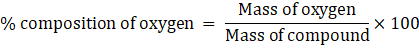⇒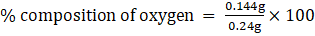⇒ % composition of oxygen = 0.6g × 100⇒ % composition of oxygen = 60%To calculate the percent of the composition of boron, we apply the formula: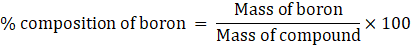⇒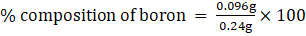⇒ % composition of boron = 0.4g × 100⇒ % composition of boron = 40%Total percentage composition of compound is 60% + 40% = 100%Question 6.In a class, a teacher asked to write the molecular formula of oxygen Shamita wrote the formula as O2 and Priyanka as O. which one is correct? State the reason.Answer:Shamita wrote the correct formula of oxygen as O2 because:i. A molecule of oxygen has two atoms.ii. It is diatomic in nature (atomicity = 2)iii. Thus, writing oxygen as O2 indicates two separate atoms of oxygen.Note: Atomicity is the number of atoms constituting a molecule.Question 7.Imagine what would happen if we do not have standard symbols for elements?Answer:if we do not have standard symbols for elements, then it will take a lot of time to write the full name of the elements and compounds every time to describe a reaction.Question 8.Mohith said "H2 differs from 2H". Justify.Answer: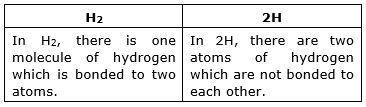Question 9.Lakshmi gives a statement "CO and Co both represents element". Is it correct? State reason.Answer:No, CO does not represent element whereas Co represents element because the first letter of the symbol is always the upper case and the second letter is always lower case.In CO, the second letter is uppercase whereas in Co, the second letter is lowercase. Hence Co represents an element.Question 10.The formula of the water molecule is H2O. What information you get from this formula.Answer:To get the information, first, we will see the elements present in a molecule of the compound.Second, we will count the number of atoms of each element of that molecule.In H2O:i. 2 atoms of hydrogen and one atom of oxygen are present.ii. There are total three atoms present in a molecule of water.Question 11.How would you write 2 molecules of oxygen and 5 molecules of Nitrogen.Answer:For oxygen:⇒ First, write the symbol of oxygen –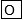⇒ Now write 2 as a subscript after O –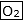For nitrogen:⇒ First, write the symbol of nitrogen –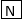⇒ Now write 5 as a subscript after N –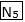Question 12.The formula of a metal oxide is MO. Then write the formula of its chloride.Answer:The formula of its chloride is MOCl2Question 13.Formula of calcium hydroxide is Ca (OH)2 and zinc phosphate is Zn3(PO4)2. Then write the formula to calcium phosphate.Answer:Valency of Ca = 2Valency of PO4 = 3Now, apply the criss cross method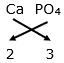So the formula will be Ca3(PO4)2Question 14.Find out the chemical names and formulae for the following common household substances.a) common saltb) baking sodac) washing sodad) vinegarAnswer:a) Common saltChemical name – sodium chlorideChemical formula – NaClb) baking sodaChemical name – sodium bicarbonateChemical formula – NaHCO3c) washing sodaChemical name – sodium carbonateChemical formula – Na2CO3d) vinegarChemical name – acetic acidChemical formula – CH3COOHQuestion 15.Calculate the mass of the following0.5 mole of N2 gas.Answer:0.5 mole of N2 gasFirst, we apply the formula: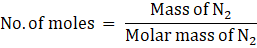⇒ Mass of N2 = No. of moles × Molar mas of N2⇒ Mass of N2 = 0.5 mole × 2(atomic mass of nitrogen)⇒ Mass of N2 = 0.5 × 2 × 14⇒ Mass of N2 = 14gThus, the mass of N2 gas is 14g.Question 16.Calculate the mass of the following0.5 mole of N atoms.Answer:0.5 mole of NatomsFirst, we apply the formula: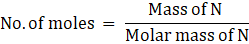⇒ Mass of Natoms = No. of moles × Molar mass of N⇒ Mass of Natoms = 0.5 mole × (atomic mass of nitrogen)⇒ Mass of Natoms = 0.5 × 14⇒ Mass of N2 = 7gThus, the mass of N atoms is 7g.Question 17.Calculate the mass of the following3.011 × 1023 number of N atoms.Answer:3.011 X 1023 number of N atoms.As we know that 1 mole = 6.022 X 1023atoms⇒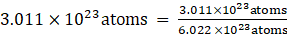⇒ 1/2 mole = 0.5 moleFor 0.5 mole of NatomsFirst, we apply the formula:⇒ Mass of Natoms = No. of moles × Molar mass of N⇒ Mass of Natoms = 0.5 mole × (atomic mass of nitrogen)⇒ Mass of Natoms = 0.5 × 14⇒ Mass of N atoms = 7gThus, mass of3.011 × 1023 number of N atoms is 7gQuestion 18.Calculate the mass of the following6.022 × 1023 number of N2 molecules.Answer:6.022 X 1023 number of N2 molecules.As we know that 1 mole = 6.022 X 1023atomsFor 1 mole of N2molecules:First, we apply the formula: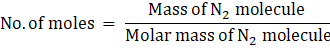⇒ Mass of N2 = No. of moles × Molar mass of N2⇒ Mass of N2 = 1 mole × 2(atomic mass of nitrogen)⇒ Mass of N2 = 1mole × 28u⇒ Mass of N2 = 28gThus, mass of6.022 × 1023 number of N2 molecules is 28g.Question 19.Calculate the number of particles in each of the following46g of NaAnswer:The number of particles present in one mole of any substance is has a fixed value of 6.022 × 1023. This number is called Avogadro constant(NA).Mass of Na = 46gMolar mass of Na = 23uApply the formula given below:Number of particles = number of moles × 6.022 × 1023⇒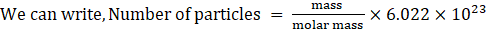⇒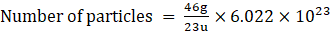⇒ Number of particles = 2 × 6.022 × 1023⇒ Number of particles = 12.046 × 1023Thus, 46g of Na contains 12.046 × 1023 particles.Question 20.Calculate the number of particles in each of the following8g of O2Answer:The number of particles present in one mole of any substance is has a fixed value of 6.022 × 1023. This number is called Avogadro constant(NA).Mass of O2 = 8gMolar mass of O2 = 32uApply the formula given below:Number of particles = number of moles × 6.022 × 1023⇒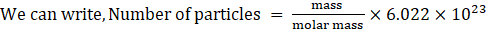⇒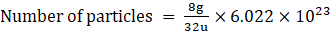⇒ Number of particles = 0.25 × 6.022 × 1023⇒ Number of particles = 1.5 × 1023Thus, 8g of O2 contains 1.5 × 1023 particles.Question 21.Calculate the number of particles in each of the following0.1 mole of hydrogenAnswer:The number of particles present in one mole of any substance is has a fixed value of 6.022 × 1023. This number is called Avogadro constant(NA).Number of mole of hydrogen = 0.1 moleMolar mass of H2 = 2uApply the formula given below:Number of particles = number of moles × 6.022 × 1023⇒ Number of particles = 0.1 mole × 6.022 × 1023⇒ Number of particles = 6.022 × 1024Thus, 0.1 mole of hydrogen contains 6.022 × 1024particles.Question 22.Convert into mole12g of O2 gas.Answer:Mass of O2 gas = 12gMolar mass of O2 = 32uApply the formula: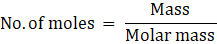⇒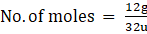⇒ No. of moles = 0.37Thus, the 12g of O2 gas is 0.37 moleQuestion 23.Convert into mole20g of water.Answer:Mass of water(H2O) = 20gMolar mass of H2O = 18uApply the formula:⇒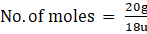⇒ No. of moles = 1.11Thus, the 20g of water is 1.11 moleQuestion 24.Convert into mole22g of carbon dioxide.Answer:Mass of CO2 gas = 22gMolar mass of CO2 = 44uApply the formula:⇒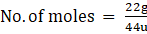⇒ No. of moles = 0.5Thus, the 22g of CO2 gas is 0.5 moleQuestion 25.Write the valencies of Fe in FeCl2 and FeCl3Answer:In FeCl2, the valency of Fe is + 2In FeCl3, the valency of Fe is + 3Question 26.Calculate the molar mass of Sulphuric acid (H2SO4) and glucose (C6H12O6)Answer:Molar mass of sulphuric acid (H2SO4):⇒ 2(atomic mass of hydrogen) + (atomic mass of Sulphur) + 4(atomic mass of oxygen)⇒ 2 × 1 + 32 + 4 × 16⇒ 2 + 32 + 64⇒ 98 uThus, the molar mass of H2SO4 is 98 u.Molar mass of glucose (C6H12O6):⇒ 6(atomic mass of carbon) + 12(atomic mass of hydrogen) + 6(atomic mass of oxygen)⇒ 6 × 12 + 12 × 1 + 6 × 16⇒ 72 + 12 + 96⇒ 108 uThus, the molar mass of C6H12O6 is 108 u.Question 27.Which has more number of atoms - 100g of sodium or 100g of iron? Justify your answer. (atomic mass of sodium = 23u, atomic mass of iron = 56u)Answer:For sodiumGiven: Mass of sodium = 100gMolar mass of sodium = 23uNumber of atoms = number of moles × 6.022 × 1023⇒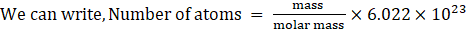⇒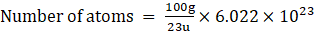⇒ Number of atoms = 4.34 × 6.022 × 1023⇒ Number of atomss = 2.613 × 1024⇒Thus, 100g of sodium contains 2.613 × 1024 atoms.For ironGiven: Mass of iron = 100gMolar mass of iron = 55.8uNumber of atoms = number of moles × 6.022 × 1023⇒⇒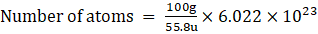⇒ Number of atoms = 1.79× 6.022 × 1023⇒ Number of atoms = 1.07× 1024⇒Thus, 100g of iron contains 1.07× 1024atoms.Therefore, 100g of sodium has more number of atoms.Question 28.Complete the following table.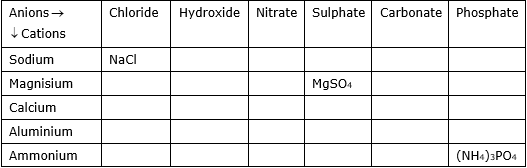Answer: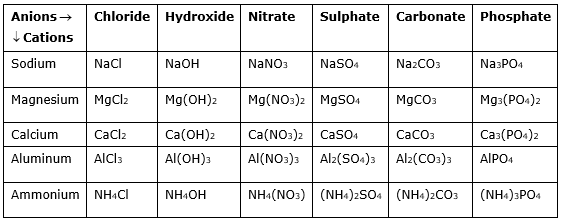Question 29.Fill the following table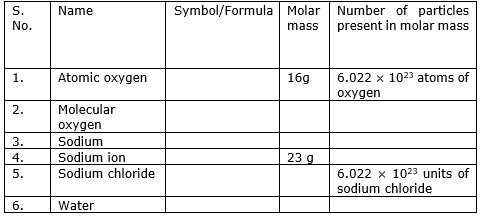Answer: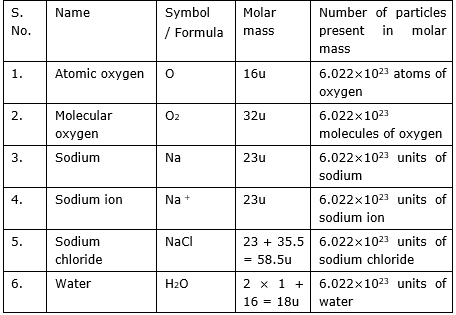PDF FILE TO YOUR EMAIL IMMEDIATELY PURCHASE NOTES & PAPER SOLUTION. @ Rs. 50/- each (GST extra)

HINDI ENTIRE PAPER SOLUTION

MARATHI PAPER SOLUTION

SSC MATHS I PAPER SOLUTION

SSC MATHS II PAPER SOLUTION

SSC SCIENCE I PAPER SOLUTION

SSC SCIENCE II PAPER SOLUTION

SSC ENGLISH PAPER SOLUTION

SSC & HSC ENGLISH WRITING SKILL

HSC ACCOUNTS NOTES

HSC OCM NOTES

HSC ECONOMICS NOTES

HSC SECRETARIAL PRACTICE NOTES

# 2019 Board Paper Solution

HSC ENGLISH SET A 2019 21st February, 2019

HSC ENGLISH SET B 2019 21st February, 2019

HSC ENGLISH SET C 2019 21st February, 2019

HSC ENGLISH SET D 2019 21st February, 2019

SECRETARIAL PRACTICE (S.P) 2019 25th February, 2019

HSC XII PHYSICS 2019 25th February, 2019

CHEMISTRY XII HSC SOLUTION 27th, February, 2019

OCM PAPER SOLUTION 2019 27th, February, 2019

HSC MATHS PAPER SOLUTION COMMERCE, 2nd March, 2019

HSC MATHS PAPER SOLUTION SCIENCE 2nd, March, 2019

SSC ENGLISH STD 10 5TH MARCH, 2019.

HSC XII ACCOUNTS 2019 6th March, 2019

HSC XII BIOLOGY 2019 6TH March, 2019

HSC XII ECONOMICS 9Th March 2019

SSC Maths I March 2019 Solution 10th Standard11th, March, 2019

SSC MATHS II MARCH 2019 SOLUTION 10TH STD.13th March, 2019

SSC SCIENCE I MARCH 2019 SOLUTION 10TH STD. 15th March, 2019.

SSC SCIENCE II MARCH 2019 SOLUTION 10TH STD. 18th March, 2019.

SSC SOCIAL SCIENCE I MARCH 2019 SOLUTION20th March, 2019

SSC SOCIAL SCIENCE II MARCH 2019 SOLUTION, 22nd March, 2019

XII CBSE - BOARD - MARCH - 2019 ENGLISH - QP + SOLUTIONS, 2nd March, 2019

# HSCMaharashtraBoardPapers2020

(Std 12th English Medium)

HSC ECONOMICS MARCH 2020

HSC OCM MARCH 2020

HSC ACCOUNTS MARCH 2020

HSC S.P. MARCH 2020

HSC ENGLISH MARCH 2020

HSC HINDI MARCH 2020

HSC MARATHI MARCH 2020

HSC MATHS MARCH 2020

# SSCMaharashtraBoardPapers2020

(Std 10th English Medium)

English MARCH 2020

HindI MARCH 2020

Hindi (Composite) MARCH 2020

Marathi MARCH 2020

Mathematics (Paper 1) MARCH 2020

Mathematics (Paper 2) MARCH 2020

Sanskrit MARCH 2020

Sanskrit (Composite) MARCH 2020

Science (Paper 1) MARCH 2020

Science (Paper 2)

Geography Model Set 1 2020-2021

MUST REMEMBER THINGS on the day of Exam

Are you prepared? for English Grammar in Board Exam.

Paper Presentation In Board Exam

How to Score Good Marks in SSC Board Exams

Tips To Score More Than 90% Marks In 12th Board Exam

How to write English exams?

How to prepare for board exam when less time is left

How to memorise what you learn for board exam

No. 1 Simple Hack, you can try out, in preparing for Board Exam

How to Study for CBSE Class 10 Board Exams Subject Wise Tips?

JEE Main 2020 Registration Process – Exam Pattern & Important Dates

NEET UG 2020 Registration Process Exam Pattern & Important Dates

How can One Prepare for two Competitive Exams at the same time?

8 Proven Tips to Handle Anxiety before Exams!